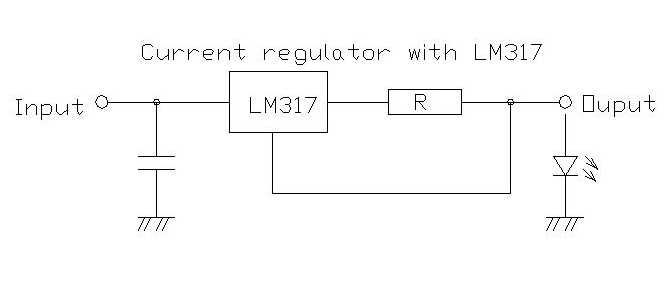# Simple constant current driver

I am searching for a cheap constant current driver/circuit. I have four of this kind that takes 12V input voltage and gives 900mA at 12V (unfortunately it is not working with 5V input).

I need a circuit that takes 5V input (from a 5V/20A power supply) and produces around 1A constant current.
Is there such a device out there? Which parts would I use to build one on my own? Thanks.

Google "constant current schematic" for several possible DIY circuits.

Could parallel three AMC7135's. Those are cheap, dropout can be as low as like 0.1~0.2v, and 350mA each, approx.

paigioph:
I am searching for a cheap constant current driver/circuit. I have four of this kind that takes 12V input voltage and gives 900mA at 12V (unfortunately it is not working with 5V input).

I need a circuit that takes 5V input (from a 5V/20A power supply) and produces around 1A constant current.
Is there such a device out there? Which parts would I use to build one on my own? Thanks.

You will need to step up the 5 volts to 12 (or more) volts. Then, use this classic circuit:The constant current is defined by R and the formula is `[b]R = (1.2 / I)[/b]`

So, for 900 mA, you would do R = 1.2 / 0.9 = 1.33 ohms.

Of course, for 12 volt compliance voltage, you need at least 2 to 3 volts more on the input, so your 5 volt up-converter should provide 15 volts DC minimum.

Downside of that ancient circuit is that it has a dropout voltage of ~3.25volt@1Amp
2volt across the regulator and 1.25volt across the resistor.
Not possible with this circuit to power a ~3.3volt LED from 5volt.
Leo..

I've used this circuit to hold 1.5 amps through an E-cig heater, worked very well, if load is non inductive (resistance or LED) you won't need D1, though you may need to heat sink Q1.0.5V on pot puts 1 amp thru load.

You may have to be careful about power dissipation in the power device - it will have to dissipate

(Vin - Vout)* current drawn

watts.

A heatsink may well be required.

regards

Allan